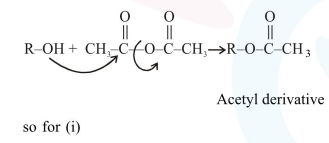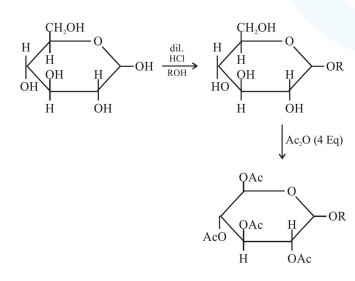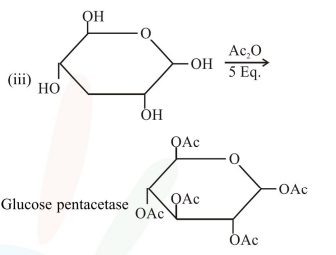# Consider the following reactions :

Question:

Consider the following reactions :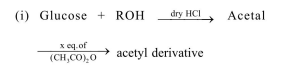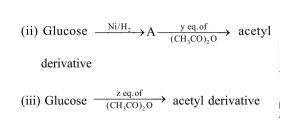' $x$ ', ' $y$ ' and ' $z$ ' in these reactions are respectively.

1. $5,6, \& 5$

2.  $4,5 \& 5$

3. $5,4 \& 5$

4. $4,6 \& 5$

Correct Option: , 4

Solution: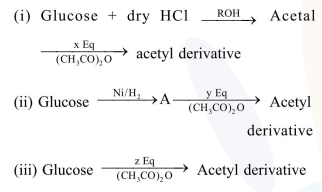due to presence of - OH group in Glucose the reaction is